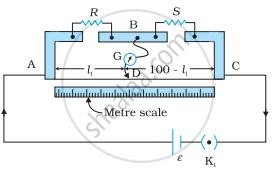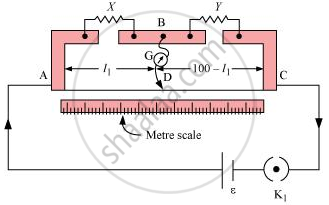# (a) In a metre bridge [shown in the figure], the balance point is found to be at 39.5 cm from the end A, when the resistor Y is 12.5 Ω. - Physics

Numerical

(a) In a metre bridge [shown in the figure], the balance point is found to be at 39.5 cm from the end A, when the resistor Y is 12.5 Ω. Determine the resistance of X. Why are the connections between resistors in a Wheatstone or meter bridge made of thick copper strips?

(b) Determine the balance point of the bridge above if X and Y are interchanged.

(c) What happens if the galvanometer and cell are interchanged at the balance point of the bridge? Would the galvanometer show any current?#### Solution

A metre bridge with resistors X and Y is represented in the given figure.(a) Balance point from end A, l1 = 39.5 cm

Resistance of the resistor Y = 12.5 Ω

Condition for the balance is given as,

"X"/"Y" = (100 - "l"_1)/("l"_1)

X = (100 - 39.5)/39.5 xx 12.5 = 8.2 Ω

Therefore, the resistance of resistor X is 8.2 Ω.

The connection between resistors in a Wheatstone or metre bridge is made of thick copper strips to minimize the resistance, which is not taken into consideration in the bridge formula.

(b) If X and Y are interchanged, then l1 and 100 − l1 get interchanged.

The balance point of the bridge will be 100 − l1 from A.

100 − l= 100 − 39.5 = 60.5 cm

Therefore, the balance point is 60.5 cm from A.

(c) When the galvanometer and cell are interchanged at the balance point of the bridge, the galvanometer will show no deflection. Hence, no current would flow through the galvanometer.

Concept: Metre Bridge
Is there an error in this question or solution?

#### APPEARS IN

NCERT Physics Part 1 and 2 Class 12
Chapter 3 Current Electricity
Exercise | Q 3.10 | Page 128
NCERT Class 12 Physics Textbook
Chapter 3 Current Electricity
Exercise | Q 10 | Page 128

Share